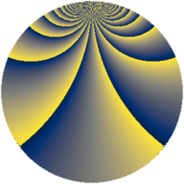# Properties

 Label 900.3.qLevel $900$ Weight $3$ Character orbit 900.q Rep. character $\chi_{900}(499,\cdot)$ Character field $\Q(\zeta_{6})$ Dimension $424$ Sturm bound $540$

# Related objects

## Defining parameters

 Level: $$N$$ $$=$$ $$900 = 2^{2} \cdot 3^{2} \cdot 5^{2}$$ Weight: $$k$$ $$=$$ $$3$$ Character orbit: $$[\chi]$$ $$=$$ 900.q (of order $$6$$ and degree $$2$$) Character conductor: $$\operatorname{cond}(\chi)$$ $$=$$ $$180$$ Character field: $$\Q(\zeta_{6})$$ Sturm bound: $$540$$

## Dimensions

The following table gives the dimensions of various subspaces of $$M_{3}(900, [\chi])$$.

Total New Old
Modular forms 744 440 304
Cusp forms 696 424 272
Eisenstein series 48 16 32

## Trace form

 $$424q + 2q^{4} - 12q^{6} + 8q^{9} + O(q^{10})$$ $$424q + 2q^{4} - 12q^{6} + 8q^{9} + 18q^{14} - 2q^{16} - 28q^{21} - 86q^{24} - 88q^{26} - 44q^{29} - 2q^{34} + 240q^{36} - 4q^{41} - 504q^{44} + 144q^{46} - 1312q^{49} + 374q^{54} + 12q^{56} - 4q^{61} + 272q^{64} - 398q^{66} + 396q^{69} - 284q^{74} - 126q^{76} - 408q^{81} + 142q^{84} - 210q^{86} - 464q^{89} + 138q^{94} - 972q^{96} + O(q^{100})$$

## Decomposition of $$S_{3}^{\mathrm{new}}(900, [\chi])$$ into newform subspaces

The newforms in this space have not yet been added to the LMFDB.

## Decomposition of $$S_{3}^{\mathrm{old}}(900, [\chi])$$ into lower level spaces

$$S_{3}^{\mathrm{old}}(900, [\chi]) \cong$$ $$S_{3}^{\mathrm{new}}(180, [\chi])$$$$^{\oplus 2}$$